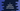# typedef in C explanation with examples## typedef in C with examples:

typedef is a keyword in C that is used to create an alternate name for an existing datatype.

For example, if you want to use a different name for int, you can use typedef to set it any other name before the program is written. Inside the program, you can use the new name instead of int.

We can use typedef with user-defined data types like structure, union as well.

In this post, I will show you how typedef works with different examples.

### Definition of typedef:

typedef is defined as like below:

``typedef existing_type_name alias_name``

Here, existing_type_name is the existing type and alias_name is the new name.

### Example of typedef with int:

Let’s take a look at the below example:

``````#include <stdio.h>

typedef int n;

int main()
{
n i;

printf("Enter a value: ");
scanf("%d", &i);

printf("You have entered %d\n", i);
}``````

In this program,

• We are using typedef to define int as n.
• The variable i is initialized as n i, that will act like int i.
• We are using i similar to any other integer to read and write.

It will print output as like below:

``````Enter a value: 12
You have entered 12``````

### Example of typedef with unsigned int:

typedef is useful to create alias for long data types. For example, we can use it for unsigned int, unsigned long etc. to create a short name.

``````#include <stdio.h>

typedef unsigned int uInt;

int main()
{
uInt i;

printf("Enter a value: ");
scanf("%u", &i);

printf("You have entered %u\n", i);
}``````

It will give similar output.

``````Enter a value: 33
You have entered 33``````

### Example of typedef with pointers:

We can use typedef with pointer variables. For example:

``````#include <stdio.h>

typedef int *intP;

int main()
{
intP p;
int i = 10;

p = &i;

printf("Value %d\n", *p);
}``````

We are using typedef to define integer pointer as intP.

### Example of typedef with structure:

typedef is pretty useful with structure and unions. We can use it to create a name for a structure and then create variables using that name.

For example:

``````#include <stdio.h>

typedef struct
{
char name;
int age;
} Student;

int main()
{
Student s;

printf("Enter the name of the student: ");
scanf("%s", s.name);

printf("Enter the age of the student: ");
scanf("%d", &s.age);

printf("Name: %s, Age: %d\n", s.name, s.age);
}``````

In this program, we created a structure and named it as Student. A variable s is created and the name and age are read from the user as input. The last line is printing the content.

It will print output as like below:

``````Enter the name of the student: Alex
Enter the age of the student: 20
Name: Alex, Age: 20``````